# Stable Maps and Unfoldings

## Functions of one variable

Lets study the simplest case: Maps from $\mathbb R$ to $\mathbb R$. Here we have a much simpler set of singularities which just consist of the A-series:### A0

• Normal form $f(x) = x$### A1

• Normal form $f(x) = x^2$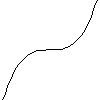### A2

• Normal form $f(x) = x^3$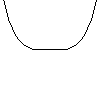### A3

• Normal form $f(x) = x^4$

We notice in this case how the type of singularity depends on how many derivatives vanish. This is one of the roots of singularity theory, how can you generalise the classification of functions interms of vanishing defivatives to general maps from $\mathbb R^n$ to $\mathbb R^p$ ? As we have seen in the previous section this is not entirly straight forward. For example we get the D and E series when we have two or more variables this does not occur for functions of one variable.

## Deforming the functions

Now what happens if we slightly perterb the function. If we add an extra term term to the function, say replacing $x^3$ by $x^3 + a x$ we get two cases: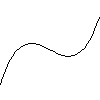$f(x) = x^3-\tfrac{1}{2} x$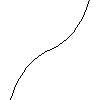$f(x) = x^3+\tfrac{1}{2} x$

In the first we have two A1 singularities the max and min but in the second there are no singularities. We say that the A2 two point is un-stable as it normally vanishes when we disturb the function.

On the otherhand an A1 singularity is stable as we can add a small multiple of any function and we will still get and A2 singularity. For example we could have $x^2+a x$ or $x^2 + a x^3$ and for suficiently small values of a there will be a point near the origin which is an A1 singularity.$f(x) = x^2 + x$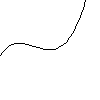$f(x) = x^2 + x^3$

Note in the second of these a second A1 point has appeared, this need not concern us here as we are only interested in the local behaviour, for very small values of a this other singularity will be a long way from the origin.

## Maps from $\mathbb R^2$ to $\mathbb R^2$

In the case of maps from $\mathbb R$ to $\mathbb R$ we only have one type of stable singularity: A1, and for maps from $\mathbb R^3$ to $\mathbb R$ none of the singularities are stable (adding $a x$ to $x^2-y^2-z^2$ splits the zero set into two smooth pieces). Things get more interesting if we study maps from the plane to the plane. Here we have two cases the fold $(x,y)\to(x,y^2)$ and the cusp $(x,y)\to(x,y^3)$. To help visulise these maps we can think of them as the composition of a map from $\mathbb R^2$ into $\mathbb R^3$ (this gives a parametrisation of a surface in three space) and projections into the plane. For the fold map we parametrise the surface by $(x,y)\to(x,y^2,y)$ and project into the plane using $(x,y,z)\to(x,y)$. The composition gives $(x,y)\to(x,y^2)$. The picture below show the surface and its projection, the projection direction is vertical, and the black lines indicate the fold points.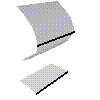### The Fold

• $(x,y)\to(x,y^2)$
• Composition of $(x,y)\to(x,y^2,y)$
• and $(x,y,z)\to(x,y)$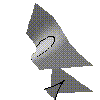### The Cusp

• $(x,y)\to(x,y^3+x y)$
• Composition of $(x,y)\to(x,y^3+x y,y)$
• and $(x,y,z)\to(x,y)$

## Maps from $\mathbb R^3$ to $\mathbb R^3$

Things get more interesting when we examine maps from 3-space to 3-space. We have the equivilent of folds and cusps (for example $(x,y,z)\to(x,y,z^2)$ and $(x,y,z)\to(x,y,z^3+y z)$) but we also get another stable map: $(x,y,z)\to(x,y,z^4+x z + y z^2)$ called the swallowtail map.

This time we will just draw the critical set, i.e. the points in the source where the map is singular, and the image of the critical set which is called the discriminant. At a typical point on the critical set the map will be locally a fold map, at some points (marked in black) the map is locally a cusp map and the image of such points forms a cispidal edge, and at the origin its of swallowtail type.

### The Swallowtail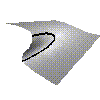#### Source

• Critical Set of the map $(x,y,z)\to(x,y,z^4+x z + y z^2)$
• i.e. $(x,y,z)$ such that $4 z^3 + x + 2 y z = 0$#### Target

• Discriminant of the map $(x,y,z)\to(x,y,z^4+x z + y z^2)$
• i.e. image of the critical set

Msqli defined